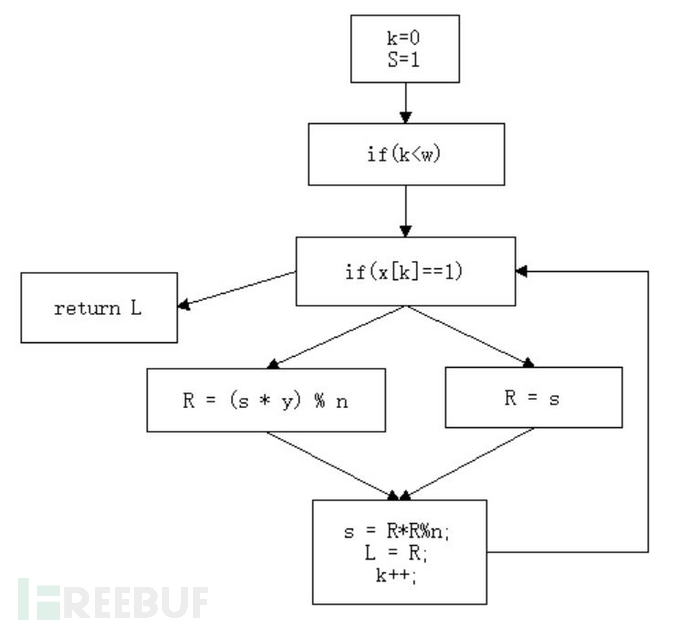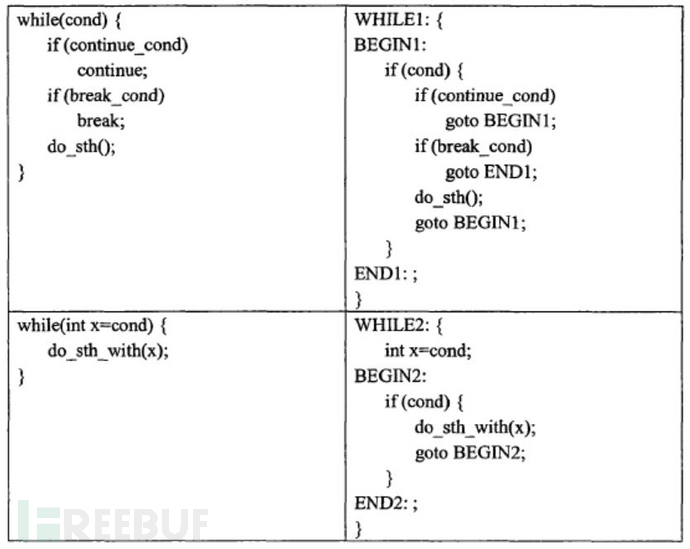# 代码混淆之道——控制流扁平与不透明谓词理论篇

int modexp(int y, int x[], int w, int n)
{
int R, L;
int k = 0;
int s = 1;
while(k < w) {
if (x[k] == 1) {
R = (s * y) % n;
}
else {
R = s;
}
s = R * R % n;
L = R;
k++;
}
return L;
}int modexp(int y, int x[], int w, int n)
{
int R, L, s, k;
int next = 0;
for(;;) {
switch(next) {
case 0: k = 0; s = 1; next = 1; break;
case 1: if(k<w) next = 2; else next = 6; break;
case 2: if(x[k]==1) next = 3; else next = 4; break;
case 3: R=(s * y) % n; next = 5; break;
case 4: R = s; next = 5; break;
case 5: s=R * R % n; L = R; k++; next = 1; break;
case 6: return L;
}
}
}

//预编译
gcc -E test.c -o test.i
//编译
gcc -S test.i -o test.s
//汇编
gcc -c test.s -o test.o
//链接
ld -static test1.o test2.o tes3.o -start-group -lgcc -lgcc_eh -lc -end-group crtend.o crtn.o

1、将函数体拆分为多个基本块，构建控制流图。将这些原本属于不同层级的基本块放到同一层级；

2、将所有基本块封装到一个switch选择分支当中；

3、用一个状态变量来表示当前状态，进行逻辑顺序控制（上述代码中的next变量）。

1、由于声明提前，声明和赋值过程分离，而引用类型需要声明的同时定义，代码如下

while（k<m） {
int& a = k;     //引用需同时声明和定义
if(...) {
a += ...
}
else {
a -= ...
}
...
}

//混淆后变以下

int &a;             //错误
switch(next) {
case ...:   if (k<m) a = k;
...
case ...:   if(...) a+=...;else a-=...;next=...;
...
}

2、构造函数和析构函数会因为声明位置而产生副作用。

3、带来同名变量的问题，即原本不同作用域名称相同的变量变成同作用域名称相同的变量。

4、try-catch语句可能会遇到的执行顺序问题。

3.1标识符重命名

3.2控制语句展开3.3变量声明提前

3.4控制流压扁

对函数有控制流图cfg

count = 0

foreach node in cfg：
if node != exit：
新建一个case，并包含node的全部内容
若node有一个后继节点：
nextVar = x
x为后继节点的case
若node有两个后继节点a1，a2：
if condition：
nextVar = x
else
nextVar = y
x为a1的case，y为a2的case
增加一个break;

if ((x * x + x) % 2 == 0) {
...
}
else {
...
}

int a[] = {1, 2, 4, 12, 16...}
int i = 0;
int next = a - 1;
switch(next) {
case 0: ...; next = a[i + 1] - a[i]; i++; break;
case 1: ...; next = a[i + 2] / a[i + 1]; i+=2; break;
case 2: ...; return ;
case 3: ...; next = a[i + 1] - a[i]; i--; break;
case 4: ...; next = a[i - 2] * a[i - 1]; break;
}

next为a-a进入case1；

next为a / a进入case3；

next为a – a进入case4；

next为a * a进入case2；

PS：本文部分名词解释、图片来自一下资料：

《软件加密与解密》

PSS：基于clang的混淆工具个GitHub上有一个，但是是clang3.3的，太老了，我最近在重构，版本为最新的clang3.9.1

https://github.com/penguin-wwy/cppobfuscator

git地址放出来，有想一起重构的可以一起，看热闹的可以帮我点个star，给点动力。

PSSS

posted @ 2017-08-17 17:34  i春秋学院  阅读(2170)  评论(0编辑  收藏